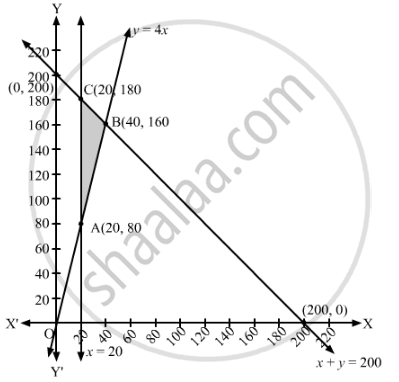# An Aeroplane Can Carry a Maximum of 200 Passengers. a Profit of ₹1000 is Made on Each Executive Class Ticket and a Profit of ₹600 is Made on Each Economy Class Ticket. - Mathematics

Sum

An aeroplane can carry a maximum of 200 passengers. A profit of ₹1000 is made on each executive class ticket and a profit of ₹600 is made on each economy class ticket. The airline reserves atleast 20 seats for executive class. However, atleast 4 times as many passengers prefer to travel by economy class than by the executive class. Determine how many tickets of each type must be sold in order to maximise the profit of the airline. What is the maximum profit?

#### Solution

Suppose x tickets of executive class and y tickets of economy class are sold by the airline.
The profit on each executive class ticket is ₹1000 and on each economy class ticket is ₹600. Therefore, the total profit from x executive class tickets and y economy class ticket is ₹(1000+ 600y).
Now, the aeroplane can carry a maximum of 200 passengers.
∴ x + y ≤ 200
The airline reserves atleast 20 seats for executive class.
∴ x ≥ 20
Also, atleast 4 times as many passengers prefer to travel by economy class than by the executive class.
∴ ≥ 4x
Thus, the given linear programming problem is
Maximise Z = 1000+ 600y
subject to the constraints
x + y ≤ 200
x ≥ 20
≥ 4x
x, y ≥ 0
The feasible region determined by the given constraints can be diagrammatically represented as,The coordinates of the corner points of the feasible region are A(20, 80), B(40, 160) and C(20, 180).

The value of the objective function at these points are given in the following table.

 Corner Point Z = 1000x + 600y (20, 80) 1000 × 20 + 600 × 80 = 68000 (40, 160) 1000 × 40 + 600 × 160 = 136000  → Maximum (20, 180) 1000 × 20 + 600 × 180 = 128000

The maximum value of Z is 136000 at x = 40, y = 160.

Hence, 40 tickets of executive class and 160 tickets of economy class should be sold to maximise the profit. The maximum profit of the airline is ₹1,36,000.

Concept: Graphical Method of Solving Linear Programming Problems
Is there an error in this question or solution?

#### APPEARS IN

RD Sharma Class 12 Maths
Chapter 30 Linear programming
Exercise 30.4 | Q 51 | Page 57